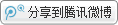用户名：
密码：###### 马雷:联合演算何以可能

(一) 词项逻辑与谓词逻辑

"x(S(x) ?P(x))；
"x(S(x) ?┐P(x))；
\$ x(S(x)∧P(x))；
\$ x(S(x)∧┐P(x))。

"x(S(x) ?P(x)) ├ ┐\$ x(S(x)∧┐P(x))

(二) 词项逻辑与类逻辑ICP证号：浙ICP备16005704号-2